io7m | single-page | multi-page | Simple GPU Outline Shaders

 IDENTIFIER 26408575-e66d-44ba-a153-9e1353c5bcae TITLE Simple GPU Outline Shaders CREATOR Mark Raynsford DATE 2022-10-29T19:10:44+00:00 LANGUAGE en RIGHTS Public Domain

# 1. Overview

This document describes a simple algorithm for producing outlines in images rendered by GPUs. The technique is low-cost and runs entirely in screen-space, and can be fitted into any ordinary deferred rendering pipeline. The technique was developed by a user named KTC in a post on StackExchange. This document attempts to detail how the algorithm works.

### 1.2. Example Outlines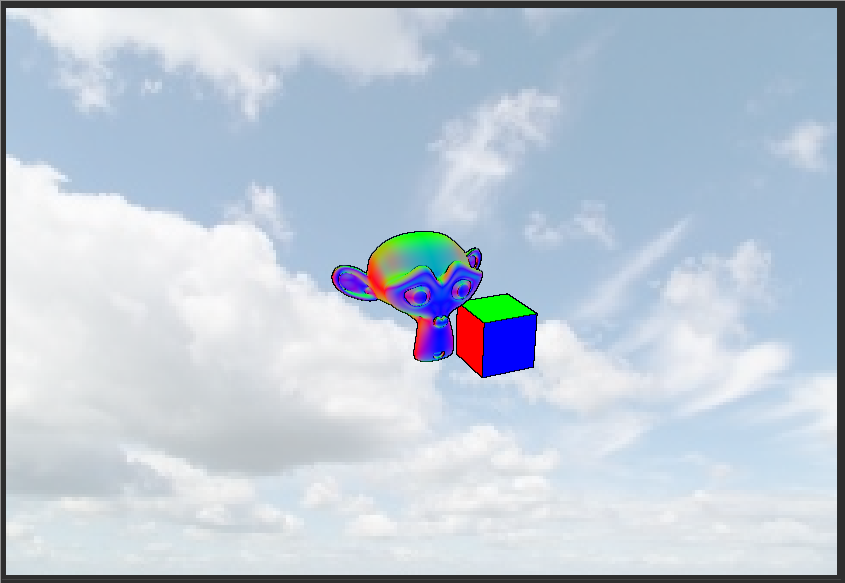# 2. Rendering Process

## 2.1. Overview

There are at least two possible techniques used to produce outlines. The first technique performs edge detection on a simple monochrome mask image. This technique is only capable of producing pure silhouette outlines on images and will not produce outlines on the edges of objects that appear inside their silhouettes.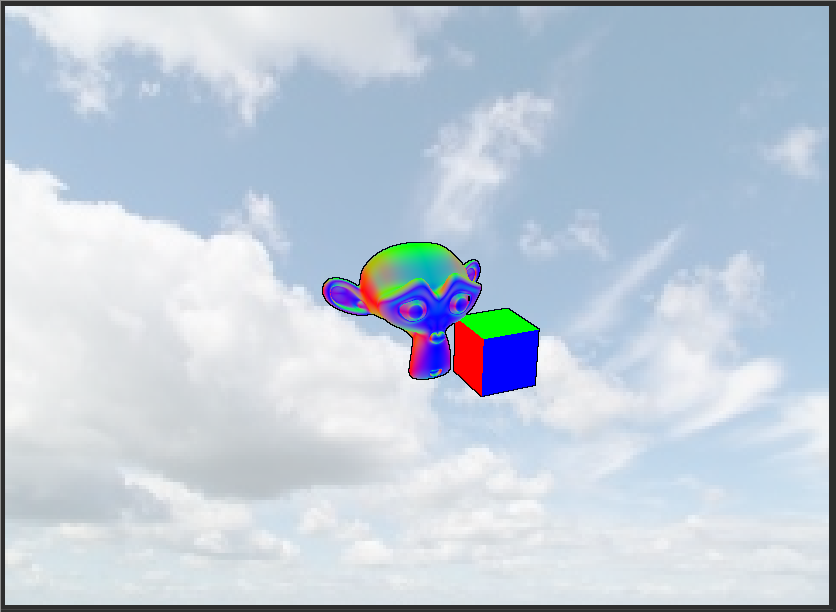The second technique performs edge detection by sampling surface normals from a rendered image. This will allow for producing outlines that appear on edges inside the silhouettes of objects, but requires data that will probably not be present in traditional forward rendering pipelines. Note the dark lines on the eyebrow regions of the face, and on the internal edges of the cube:

### 2.1.4. Outlines From Normals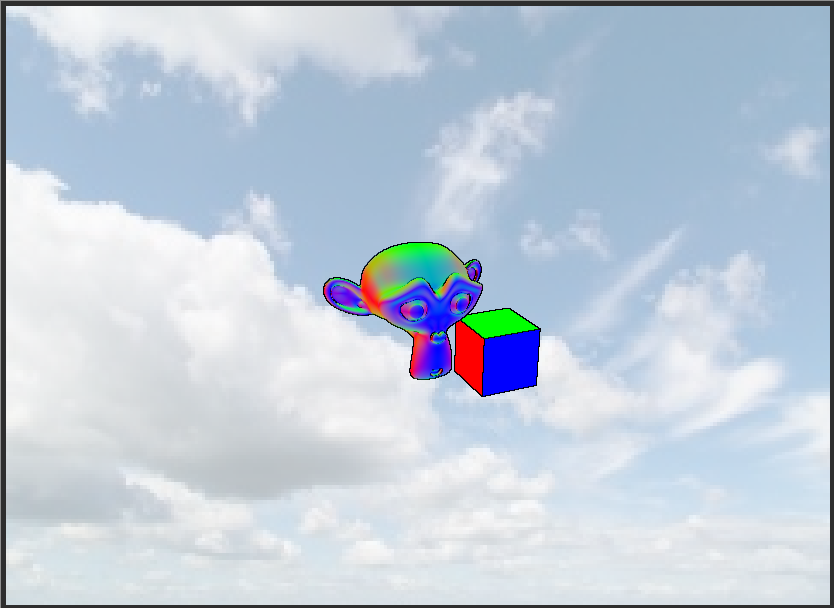First, render all the objects that should receive outlines into a monochrome image using a flat shader. The image should be initialized to 0.0, and all the pixels or fragments that make up an object should be set to 1.0.
The example scene yields the following image when using an R8-format render target: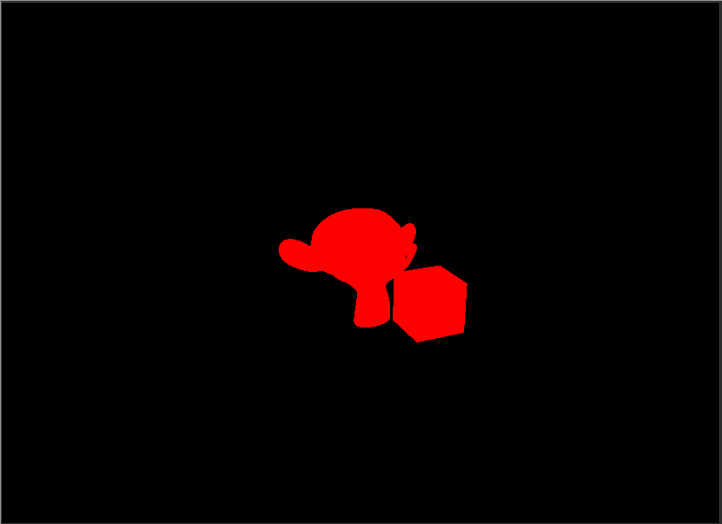Then, the rendered mask image is examined. For each pixel P in the mask image, three additional pixels are sampled from the mask: The pixel directly above P on the Y axis, the pixel directly to the right of P on the X axis, and the pixel directly above and to the right of P on the X and Y axes.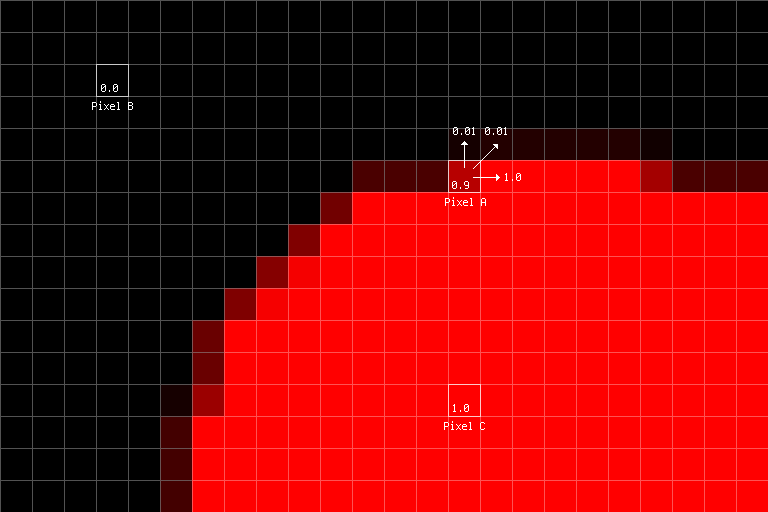In the image above, assuming that we are currently processing pixel A in the image, we can see that the pixels above A both have a value of 0.01, and the pixel directly to the right of A has a value of 1.0.
We then calculate the differences between the pixel A, and the neighbouring pixels we sampled, and take the maximum of the absolute values of these differences. This is accomplished with the following trivial GLSL code:

### 2.2.8. Differences

```float mCenter   = texture(gbufferMask, uvCenter).r;

float dT  = abs(mCenter - mTop);
float dR  = abs(mCenter - mRight);
float dTR = abs(mCenter - mTopRight);

float delta = 0.0;
delta = max(delta, dT);
delta = max(delta, dR);
delta = max(delta, dTR);```
Essentially, the resulting delta term specifies, as a real number in the range [0, 1], how likely it is that the current pixel is on the border of an object. If the delta value for each pixel is rendered to the screen, the following image will result: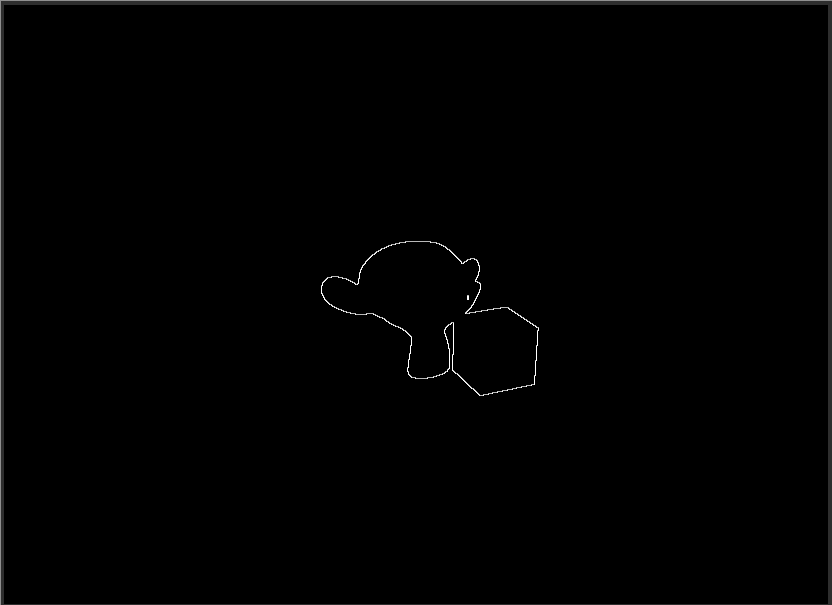Because the mask image is a monochrome image with hard edges, the outlines produced are very precise and hard-edged, and typically the delta value is exactly 0.0 or 1.0. Additionally, because the sampling occurs on pixels that are direct neighbours of the current pixel, the outlines tend to be exactly one pixel thick.
The following GLSL shader implements the full algorithm, and combines the produced outline with the albedo image to produce an image with dark outlines:

```#version 330

out vec4 fsColor;

in vec2 vsUV;

uniform vec2 viewportSize;

#define LINE_WEIGHT 1.0

uniform sampler2D gbufferNormals;
uniform sampler2D gbufferAlbedo;

void main()
{
float dx = (1.0 / viewportSize.x) * LINE_WEIGHT;
float dy = (1.0 / viewportSize.y) * LINE_WEIGHT;

vec2 uvCenter   = vsUV;
vec2 uvRight    = vec2(uvCenter.x + dx, uvCenter.y);
vec2 uvTop      = vec2(uvCenter.x,      uvCenter.y - dx);
vec2 uvTopRight = vec2(uvCenter.x + dx, uvCenter.y - dx);

float dT  = abs(mCenter - mTop);
float dR  = abs(mCenter - mRight);
float dTR = abs(mCenter - mTopRight);

float delta = 0.0;
delta = max(delta, dT);
delta = max(delta, dR);
delta = max(delta, dTR);

vec4 outline = vec4(delta, delta, delta, 1.0);
vec4 albedo  = texture(gbufferAlbedo, vsUV);

fsColor = albedo - outline;
}```
The program above uses a constant LINE_WEIGHT that will cause direct neighbouring pixels to be sampled. If this constant is set to a higher value, the resulting outlines become heavier: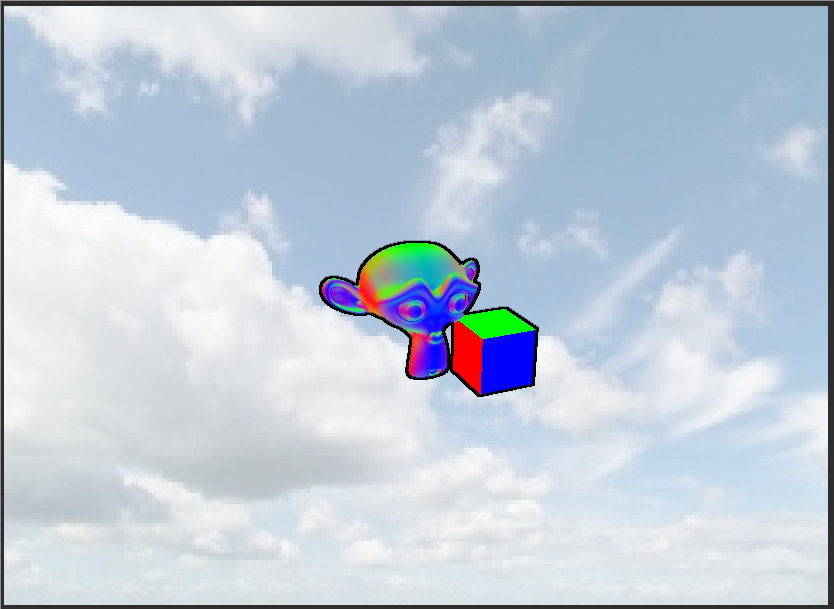As mentioned earlier, the algorithm is only capable of producing outlines on the outer edges of objects. Additionally, the algorithm requires rendering objects specifically to a separate mask image, which may be undesirable in an existing deferred rendering pipeline. We will now turn to an algorithm that can produce outlines on internal edges of objects, and can use the surface normals that are almost certainly already present in the G-buffer of any deferred rendering pipeline.

## 2.3. Outlines Using Normals

This variant of the algorithm proceeds similarly to the masking variant, except that the image that is inspected is the image that contains the surface normals for the scene instead of being a separate mask image.

### 2.3.2. Scene Normals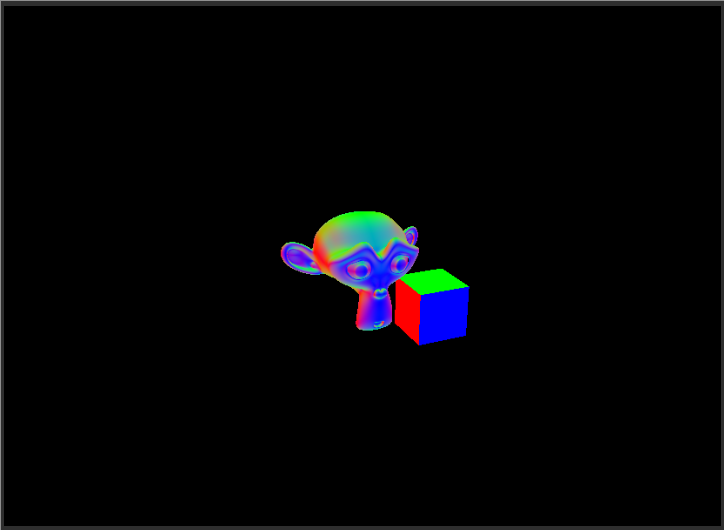The values of neighbouring pixels are sampled exactly as before, but the pixels are now three-element normal vectors instead of scalar floating point values. The delta term for each pixel is calculated by taking the absolute difference between the center pixel and each neighbouring pixel, and taking whichever is the largest of the x, y, or z components of the resulting vector. The previous scalar difference code now looks like this:

### 2.3.4. Differences (Normal)

```vec3 mCenter   = texture(gbufferNormals, uvCenter).rgb;
vec3 mTop      = texture(gbufferNormals, uvTop).rgb;
vec3 mRight    = texture(gbufferNormals, uvRight).rgb;
vec3 mTopRight = texture(gbufferNormals, uvTopRight).rgb;

vec3 dT  = abs(mCenter - mTop);
vec3 dR  = abs(mCenter - mRight);
vec3 dTR = abs(mCenter - mTopRight);

float dTmax  = max(dT.x, max(dT.y, dT.z));
float dRmax  = max(dR.x, max(dR.y, dR.z));
float dTRmax = max(dTR.x, max(dTR.y, dTR.z));

float deltaRaw = 0.0;
deltaRaw = max(deltaRaw, dTmax);
deltaRaw = max(deltaRaw, dRmax);
deltaRaw = max(deltaRaw, dTRmax);```
As surface normals tend to have somewhat soft curves, the resulting delta term, if rendered to the screen for each pixel, will tend to look like this:

### 2.3.6. Normals (Raw Delta)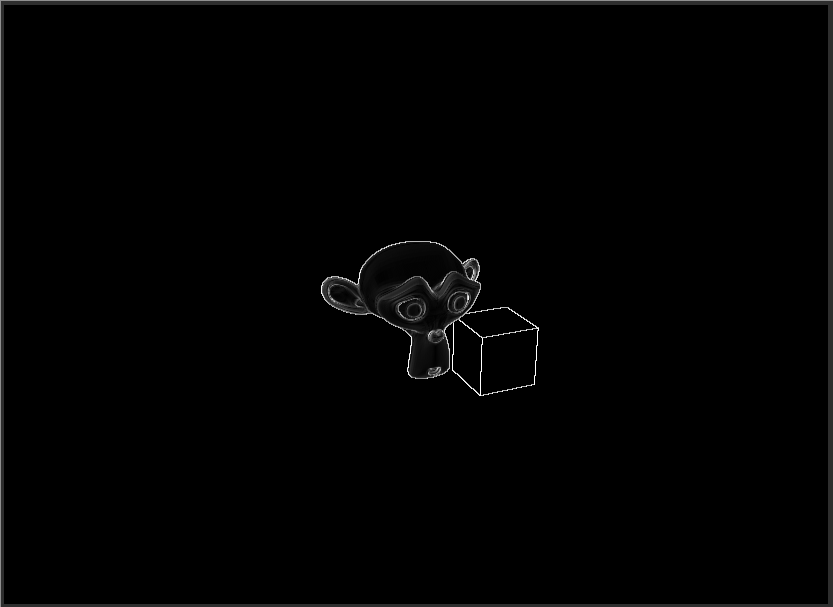This may be a desirable effect for some scenes, but if we wish to have the same hard outlines as the mask delta term, then we need to discard outlines that have an intensity below a given threshold. This is trivial to achieve by scaling and clamping the term:

### 2.3.8. Delta (Clipped)

```// Lower threshold values will discard fewer samples
// and give darker/thicker lines.
float threshold    = 0.6;
float deltaClipped = clamp((deltaRaw * 2.0) - threshold, 0.0, 1.0);```
A threshold of 0.6 will eliminate most of the soft outlines around the eyes and ears of the model, and a higher threshold of 0.8 will eliminate most of the internal edges entirely:

### 2.3.10. Normals (Clipped 0.6)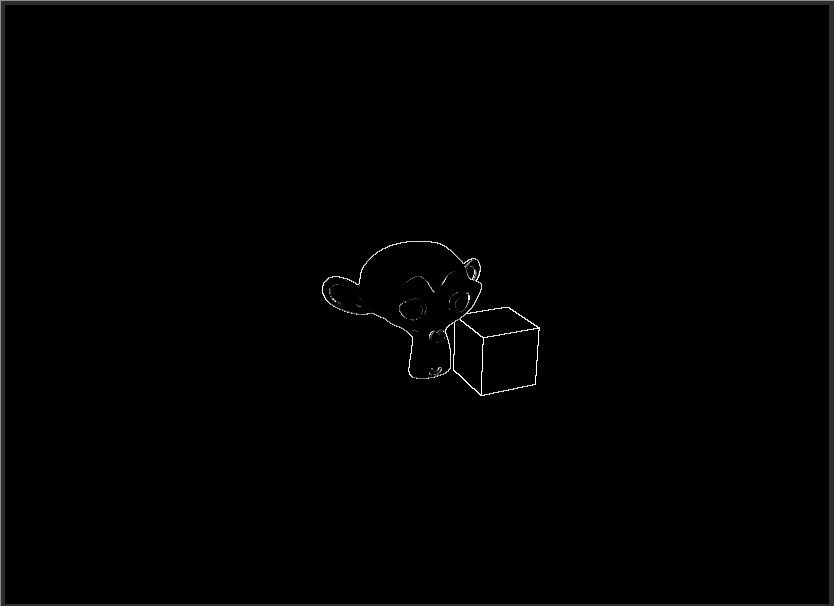### 2.3.11. Normals (Clipped 0.8)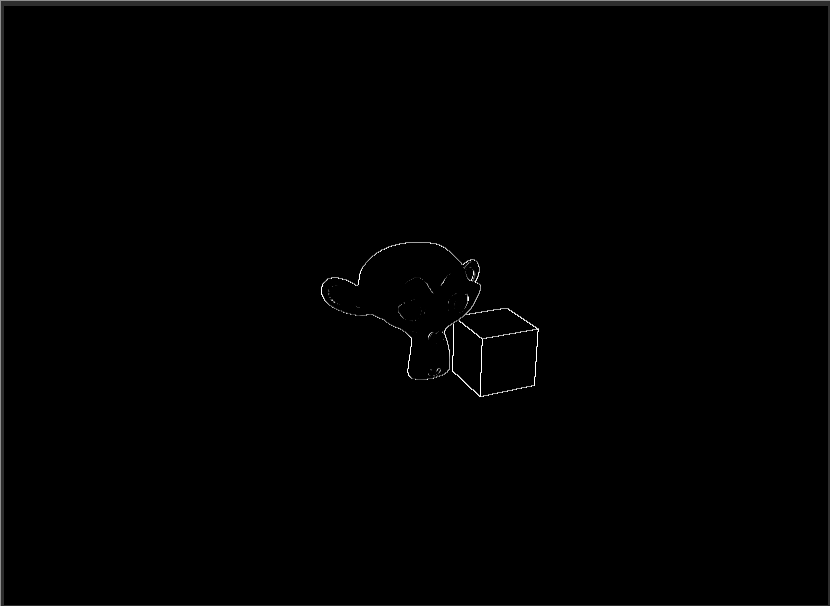The following GLSL shader implements the full algorithm, and combines the produced outline with the albedo image to produce an image with dark outlines. It provides the same LINE_WEIGHT constant that can be used to produce heavier lines.

```#version 330

out vec4 fsColor;

in vec2 vsUV;

uniform vec2 viewportSize;

#define LINE_WEIGHT 1.0

uniform sampler2D gbufferNormals;
uniform sampler2D gbufferAlbedo;

void main()
{
float dx = (1.0 / viewportSize.x) * LINE_WEIGHT;
float dy = (1.0 / viewportSize.y) * LINE_WEIGHT;

vec2 uvCenter   = vsUV;
vec2 uvRight    = vec2(uvCenter.x + dx, uvCenter.y);
vec2 uvTop      = vec2(uvCenter.x,      uvCenter.y - dx);
vec2 uvTopRight = vec2(uvCenter.x + dx, uvCenter.y - dx);

vec3 mCenter   = texture(gbufferNormals, uvCenter).rgb;
vec3 mTop      = texture(gbufferNormals, uvTop).rgb;
vec3 mRight    = texture(gbufferNormals, uvRight).rgb;
vec3 mTopRight = texture(gbufferNormals, uvTopRight).rgb;

vec3 dT  = abs(mCenter - mTop);
vec3 dR  = abs(mCenter - mRight);
vec3 dTR = abs(mCenter - mTopRight);

float dTmax  = max(dT.x, max(dT.y, dT.z));
float dRmax  = max(dR.x, max(dR.y, dR.z));
float dTRmax = max(dTR.x, max(dTR.y, dTR.z));

float deltaRaw = 0.0;
deltaRaw = max(deltaRaw, dTmax);
deltaRaw = max(deltaRaw, dRmax);
deltaRaw = max(deltaRaw, dTRmax);

// Lower threshold values will discard fewer samples
// and give darker/thicker lines.
float threshold    = 0.8;
float deltaClipped = clamp((deltaRaw * 2.0) - threshold, 0.0, 1.0);

float oI = deltaClipped;
vec4 outline = vec4(oI, oI, oI, 1.0);
vec4 albedo  = texture(gbufferAlbedo, vsUV);
fsColor = albedo - outline;
}```

All of the algorithms here are provided as a SHADERed project that can be used for experimentation.

File Description